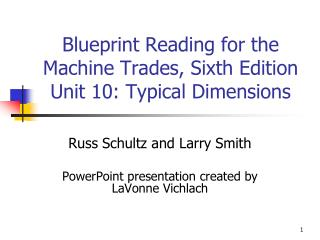DownloadDownload PresentationBlueprint Reading for the Machine Trades, Sixth Edition Unit 10: Typical Dimensions

# Blueprint Reading for the Machine Trades, Sixth Edition Unit 10: Typical Dimensions

Télécharger la présentation## Blueprint Reading for the Machine Trades, Sixth Edition Unit 10: Typical Dimensions

- - - - - - - - - - - - - - - - - - - - - - - - - - - E N D - - - - - - - - - - - - - - - - - - - - - - - - - - -
##### Presentation Transcript

1. Blueprint Reading for the Machine Trades, Sixth Edition Unit 10: Typical Dimensions Russ Schultz and Larry Smith PowerPoint presentation created by LaVonne Vichlach

2. Typical Dimensions • “TYP” means typical • Used to avoid repetitious dimensions on a drawing • E.g.—when it appears after a radial dimension-all undimensioned radii are considered to be the same • Use where repetition of same pattern is used

3. Right Triangles • Right triangles can be dimensioned by • Two sides • One side and one angle

4. Right Triangles • When angle is 45°-two sides are equal • Hypotenuse is equal to a length that is 1.414 X the length of the side

5. Repetitive Features • Repetitive features or dimensions may be specified by using an “X” after the quantity followed by the dimension • “X” designates “times”

6. Repetitive Features Examples

7. MISC DETAILS 1. How much material remains between a hole and the nearest outside edge of item #1? .189

8. MISC DETAILS 2. What is the specified material thickness ofitem 2? Brass .040

9. MISC DETAILS 3. What is the total quantity of the three items shown? 5

10. MISC DETAILS Calculate the dimensions for the letter A 1.30

11. MISC DETAILS Calculate the dimensions for the letter B 2.10

12. MISC DETAILS Calculate the dimensions for the letter C 45°

13. MISC DETAILS Calculate the dimensions for the letter D 1.8382

14. MISC DETAILS Calculate the dimensions for the letter E 2.6382

15. MISC DETAILS Calculate the dimensions for the letter F 2.30

16. MISC DETAILS Calculate the dimensions for the letter G 2.30

17. MISC DETAILS Calculate the dimensions for the letter H .70

18. MISC DETAILS Calculate the dimensions for the letter I .45

19. MISC DETAILS Calculate the dimensions for the letter J 100°

20. MISC DETAILS Calculate the dimensions for the letter K 130°

21. MISC DETAILS Calculate the dimensions for the letter L .250

22. MISC DETAILS Calculate the dimensions for the letter M 35°

23. MISC DETAILS Calculate the dimensions for the letter N 20°

24. MISC DETAILS Calculate the dimensions for the letter O 30°

25. MISC DETAILS Calculate the dimensions for the letter P .30

26. MISC DETAILS Calculate the dimensions for the letter Q .40

27. Offset Pivot BRKT 1. What type of sectional view appears on the drawing? Broken out

28. Offset Pivot BRKT • Is the material (a) nickel, or (b) molybdenum or (c) chromium alloy? (b) molybdenum

29. Offset Pivot BRKT • Is the material (a) low-, (b) medium-, or (c) high-carbon steel? (b) medium

30. Offset Pivot BRKT • How far does the boss extend above the its surrounding surface? .25

31. Offset Pivot BRKT • How far does the pad extend above the its surrounding surface? .10

32. Offset Pivot BRKT • What is the small diameter of the tapered hole? .3375

33. Offset Pivot BRKT • What is the maximum width of the slotted hole? .41

34. Offset Pivot BRKT • What is the minimum length of the slotted hole? 1.47

35. Offset Pivot BRKT • What is the minimum width of the pad? .88

36. Offset Pivot BRKT • What is the maximum length of the pad? 2.03

37. Offset Pivot BRKT Calculate the dimension for the letter: A .70

38. Offset Pivot BRKT Calculate the dimension for the letter: B 1.40

39. Offset Pivot BRKT Calculate the dimension for the letter: C 2.60

40. Offset Pivot BRKT Calculate the dimension for the letter: D .80

41. Offset Pivot BRKT Calculate the dimension for the letter: E .707

42. Offset Pivot BRKT Calculate the dimension for the letter: F .30

43. Offset Pivot BRKT Calculate the dimension for the letter: G 3.00

44. Offset Pivot BRKT Calculate the dimension for the letter: H .85

45. Offset Pivot BRKT Calculate the dimension for the letter: I 1.30

46. Offset Pivot BRKT Calculate the dimension for the letter: J 5.10

47. ADJ MTG ARM 1. What is the pitch (decimal value) of the threads? .05